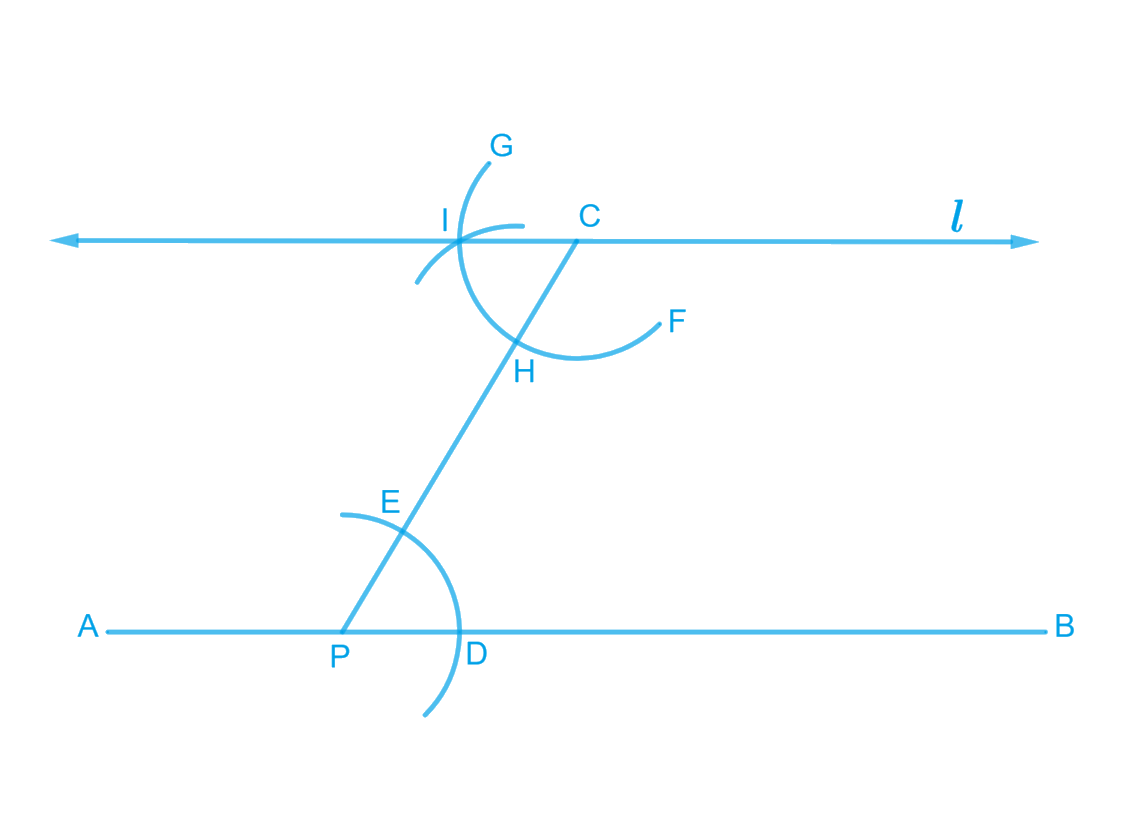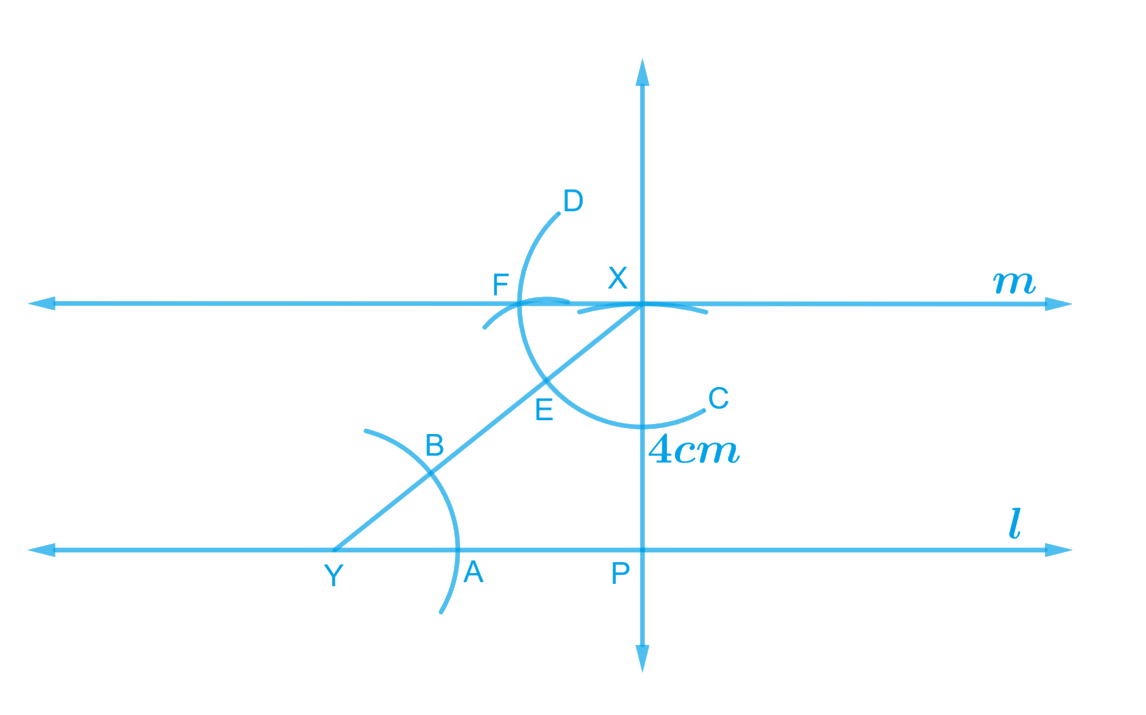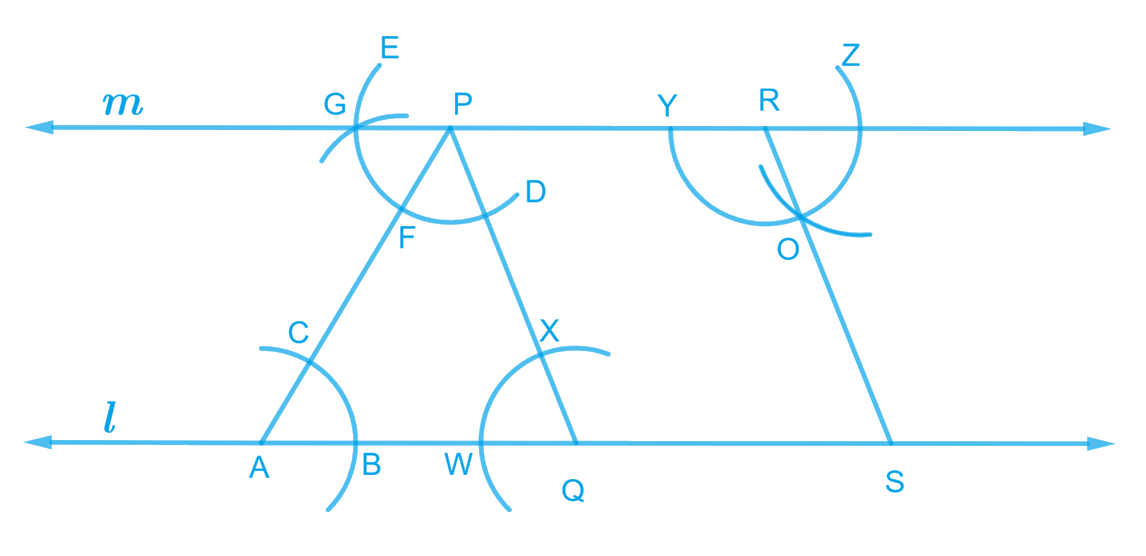# Exercise 10.1 Practical-Geometry -NCERT Solutions Class 7

Go back to  'Practical Geometry'

## Chapter 10 Ex.10.1 Question 1

Draw a line, say $$AB,$$ take a point $$C$$ outside it. Through $$C,$$draw a line parallel to $$AB$$ using ruler and compasses only.

### Solution

What is known?

A line $$AB$$ and a point $$C$$ outside it.

To construct:

A line through $$C$$ parallel to $$AB$$ using ruler and compasses.

Reasoning:

Draw a line $$AB$$ and take a point $$C$$ outside it. Draw line $$AB$$ by using ruler and compasses, follow the steps given below.

Steps:Steps of construction

1. Draw a line,  $$AB,$$ take a point $$C$$ outside this line. Take any point $$P$$ on $$AB$$. Join $$C$$ to $$P$$
2. Taking $$P$$ as centre and a convenient radius draw an arc intersecting line $$AB$$ at $$D$$ and $$PC$$ at $$E.$$
3. Taking $$C$$ as the centre and the same radius in previous step, draw an arc $$FG$$ intersecting $$PC$$ at $$H.$$
4. Adjust the compasses up to the length of $$DE$$. Without changing the opening of compasses and taking $$H$$ as the centre, draw an arc to intersect arc $$HG$$ at point $$I.$$
5. Join the point $$C$$ and $$I$$ to draw a line $$L.$$

This is the required line $$L$$ which is parallel to $$AB.$$

## Chapter 10 Ex.10.1 Question 2

Draw a line $$l$$. Draw a perpendicular to $$l$$ at any point on $$l$$. On this perpendicular choose a point $$X$$, $$4 \rm\, cm$$ away from $$l$$. Through $$X$$, draw a line $$m$$ parallel to $$l$$.

### Solution

What is known?

A line $$l$$ and $$m$$ which are $$4\,\rm{cm}$$ apart.

To construct:

A perpendicular at any point on line $$l$$, and then draw a line $$m$$ parallel to $$l$$ through $$X$$ on the perpendicular which is $$4$$ cm from the line $$l$$.

Reasoning:

Draw a line $$l$$ and then a perpendicular to $$l$$ at any point on $$l$$. On this perpendicular choose a point $$X$$, $$4$$ cm away from $$l$$. Through $$X$$, draw a line $$m$$ parallel to $$l$$. Follow the steps given below.

Steps:Steps of construction

1. Draw a line $$l$$, take a point $$P$$ on it. Draw a perpendicular passing trough point $$P.$$
2. Now adjust the compass up-to the length of $$4$$ $$\rm cm.$$ Draw an arc to intersect this perpendicular at point $$X$$ choose any point $$Y$$ on line $$l$$, join $$X$$ to $$Y$$.
3. Taking $$Y$$ as centre and with a convenient radius, draw an arc intersecting $$l$$ at $$A$$ and $$XY$$ at $$B$$.
4. Taking $$X$$ as centre and with the same radius as above, draw an arc $$CD$$ cutting $$XY$$ at $$E$$Adjust the compass up-to to the length of $$AB$$.
5. Without changing the opening of compass and taking $$E$$ as the centre, draw an arc to intersect the previously drawn arc $$CD$$ at $$F$$.
6. Join the points $$X$$ and$$F$$ to draw a line $$\rm m.$$

Line $$\rm m$$ is the required line which is parallel to $$l$$.

## Chapter 10 Ex.10.1 Question 3

Let $$l$$ be a line and$$\,P\,$$be a point not on $$l$$. Through $$P ,$$ draw a line$$\,m\,$$parallel to $$l$$. Now join$$\,P\,$$to any point $$Q$$ on $$l$$. Choose any other point $$R$$ on$$\, m \,$$. Through $$\,R,\,$$ draw a line parallel to $$PQ$$. Let this meet $$l$$ at $$S.$$ What shape do the two sets of parallel lines enclose?

### Solution

What is known?

A line $$l$$ and a point $$P$$ not on $$l$$ and line m parallel to $$l$$..

What is unknown?

Shape formed by two sets of two parallel lines.

Reasoning:

As line $$l$$ is given and$$\,P\,$$is a point not on $$l$$. Through $$P,$$ draw a line$$\,m\,$$parallel to $$l$$. Now join$$\,P\,$$to any point $$Q$$ on $$l$$. Choose any other point $$R$$ on$$\, m \,$$. Through $$\,R,\,$$ draw a line parallel to $$PQ.$$ Let this meet $$l$$ at $$S.$$

Steps:Steps of construction –

1. Draw a line $$l$$, take a point $$A$$ on it. Take a point$$\,P\,$$not on $$l$$ and join $$A$$ to $$P.$$
2. Taking $$A$$ as centre and with a convenient radius draw an arc cutting $$l$$ at $$B$$ and $$AP$$ at $$C.$$
3. Taking$$\,P\,$$as the centre and with the same radius as before, draw an arc $$DE$$ to intersect $$AP$$ at $$F.$$
4. Adjust the compasses up to the length of $$BC.$$ Without changing the opening of compasses and taking $$F$$ as the centre, draw an arc to intersect the previous drawn arc $$DE$$ at point $$G.$$
5. Join$$\,P\,$$to any point $$G$$ to draw line$$\, m \,$$. Line$$\,m\,$$will be parallel to $$l$$.
6. Join$$\,P\,$$to any point $$Q$$ on line $$l$$. Choose another point $$R$$ on line$$\, m \,$$. Similarly, a line can be drawn through point $$R$$ and parallel to $$PQ.$$
7. Let it meet line $$l$$ at point $$S.$$

In quadrilateral $$PQSR$$, opposite lines are parallel to each other.

Thus, $$PQSR$$ is a parallelogram.

Related Sections
Related Sections
Instant doubt clearing with Cuemath Advanced Math Program